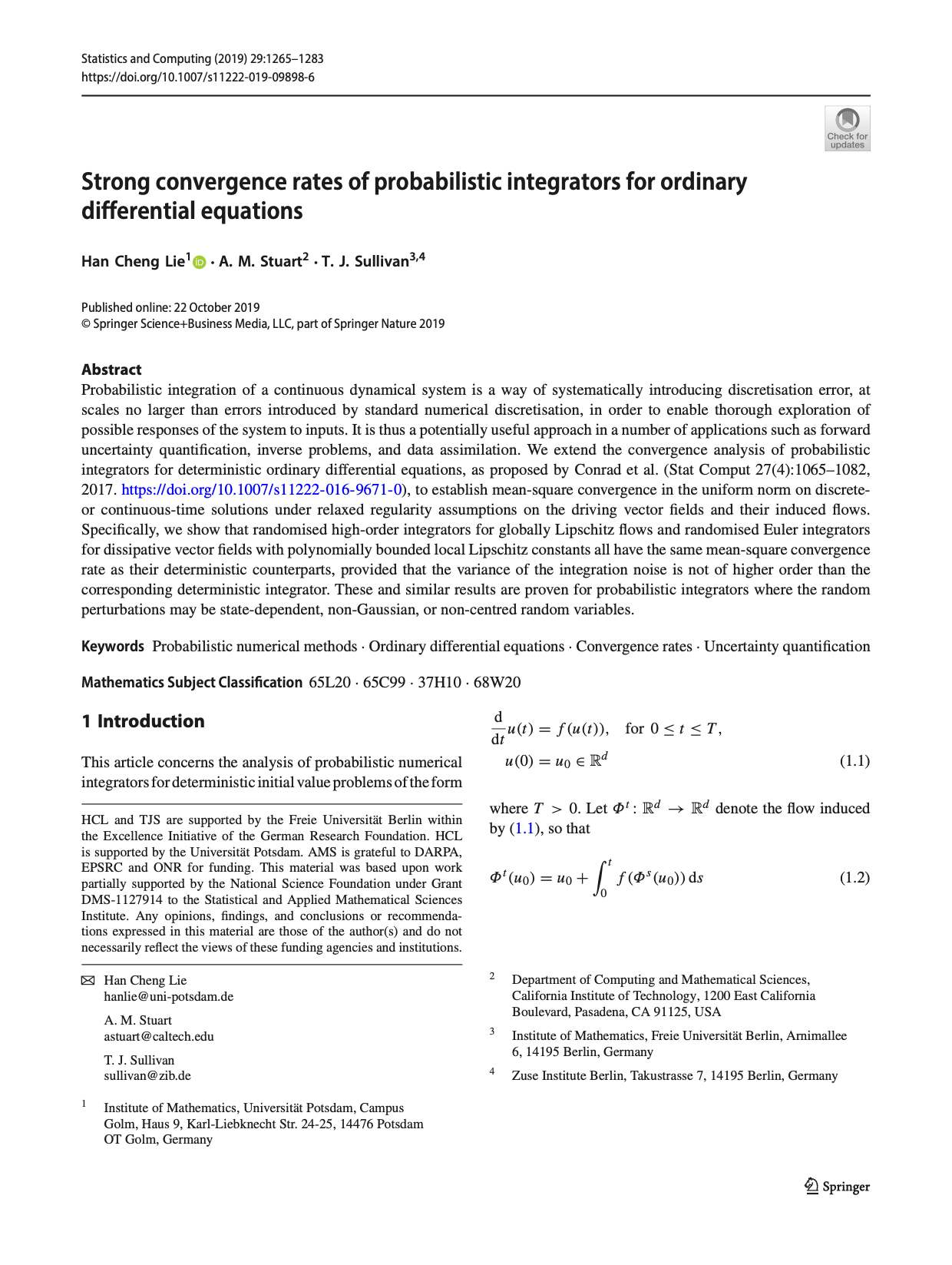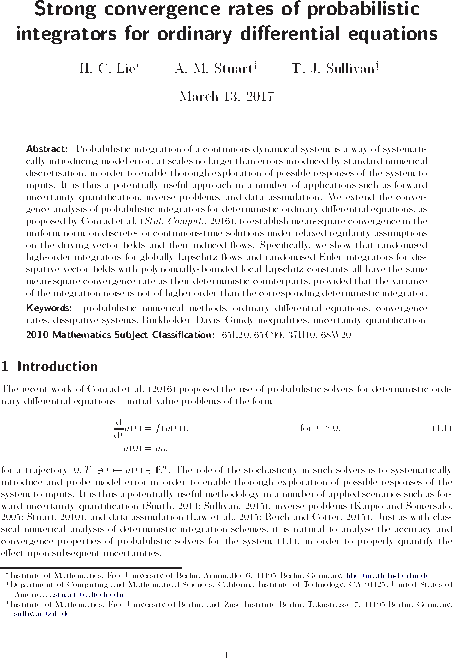# Tim Sullivan

### #stuart

Clear Search### Strong convergence rates of probabilistic integrators for ODEs in Statistics and Computing

The special issue of Statistics and Computing dedicated to probabilistic numerics carries the article “Strong convergence rates of probabilistic integrators for ODEs” by Han Cheng Lie, Andrew Stuart, and myself on the convergence analysis of randomised perturbation-based time-stepping methods for the solution of ODE initial value problems.

H. C. Lie, A. M. Stuart, and T. J. Sullivan. “Strong convergence rates of probabilistic integrators for ordinary differential equations.” Statistics and Computing 29(6):1265–1283, 2019. doi:10.1007/s11222-019-09898-6

Abstract. Probabilistic integration of a continuous dynamical system is a way of systematically introducing discretisation error, at scales no larger than errors introduced by standard numerical discretisation, in order to enable thorough exploration of possible responses of the system to inputs. It is thus a potentially useful approach in a number of applications such as forward uncertainty quantification, inverse problems, and data assimilation. We extend the convergence analysis of probabilistic integrators for deterministic ordinary differential equations, as proposed by Conrad et al. (2016), to establish mean-square convergence in the uniform norm on discrete- or continuous-time solutions under relaxed regularity assumptions on the driving vector fields and their induced flows. Specifically, we show that randomised high-order integrators for globally Lipschitz flows and randomised Euler integrators for dissipative vector fields with polynomially bounded local Lipschitz constants all have the same mean-square convergence rate as their deterministic counterparts, provided that the variance of the integration noise is not of higher order than the corresponding deterministic integrator. These and similar results are proven for probabilistic integrators where the random perturbations may be state-dependent, non-Gaussian, or non-centred random variables.

Published on Tuesday 5 November 2019 at 12:00 UTC #publication #stco #prob-num #lie #stuart### Kalman Lecture by Andrew Stuart at SFB1294

Andrew Stuart (Caltech) will give the inaugural Kalman Lecture of SFB 1294 Data Assimilation on the topic of “Large Graph Limits of Learning Algorithms”.

Time and Place. Friday 24 August 2018, 10:15–11:45, University of Potsdam, Campus Golm, Building 27, Lecture Hall 0.01

Abstract. Many problems in machine learning require the classification of high dimensional data. One methodology to approach such problems is to construct a graph whose vertices are identified with data points, with edges weighted according to some measure of affinity between the data points. Algorithms such as spectral clustering, probit classification and the Bayesian level set method can all be applied in this setting. The goal of the talk is to describe these algorithms for classification, and analyze them in the limit of large data sets. Doing so leads to interesting problems in the calculus of variations, in stochastic partial differential equations and in Monte Carlo Markov Chain, all of which will be highlighted in the talk. These limiting problems give insight into the structure of the classification problem, and algorithms for it.

Published on Friday 3 August 2018 at 11:00 UTC #event #sfb1294 #stuart### Strong convergence rates of probabilistic integrators for ODEs

Han Cheng Lie, Andrew Stuart, and I have just uploaded a preprint of our latest paper, “Strong convergence rates of probabilistic integrators for ordinary differential equations” to the arXiv. This paper is a successor to the convergence results presented by Conrad et al. (2016). We consider a generic probabilistic solver for an ODE, using a fixed time step of length $$\tau > 0$$, where the mean of the solver has global error of order $$\tau^{q}$$ and the variance of the truncation error model has order $$\tau^{1 + 2 p}$$. Whereas Conrad et al. showed, for a Lipschitz driving vector field, that the mean-square error between the numerical solution $$U_{k}$$ and the true solution $$u_{k}$$ is bounded uniformly in time as

$$\displaystyle \max_{0 \leq k \leq T / \tau} \mathbb{E} [ \| U_{k} - u_{k} \|^{2} ] \leq C \tau^{2 \min \{ p, q \}}$$

(i.e. has the same order of convergence as the underlying deterministic method), we are able to relax the regularity assumptions on the vector field / flow an obtain a stronger mode of convergence (mean-square in the uniform norm) with the same convergence rate:

$$\displaystyle \mathbb{E} \left[ \max_{0 \leq k \leq T / \tau} \| U_{k} - u_{k} \|^{2} \right] \leq C \tau^{2 \min \{ p, q \}}$$

Abstract. Probabilistic integration of a continuous dynamical system is a way of systematically introducing model error, at scales no larger than errors inroduced by standard numerical discretisation, in order to enable thorough exploration of possible responses of the system to inputs. It is thus a potentially useful approach in a number of applications such as forward uncertainty quantification, inverse problems, and data assimilation. We extend the convergence analysis of probabilistic integrators for deterministic ordinary differential equations, as proposed by Conrad et al. (Stat. Comput., 2016), to establish mean-square convergence in the uniform norm on discrete- or continuous-time solutions under relaxed regularity assumptions on the driving vector fields and their induced flows. Specifically, we show that randomised high-order integrators for globally Lipschitz flows and randomised Euler integrators for dissipative vector fields with polynomially-bounded local Lipschitz constants all have the same mean-square convergence rate as their deterministic counterparts, provided that the variance of the integration noise is not of higher order than the corresponding deterministic integrator.

Published on Monday 13 March 2017 at 13:00 UTC #preprint #prob-num #lie #stuart